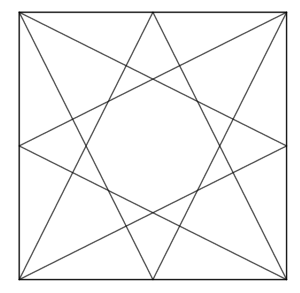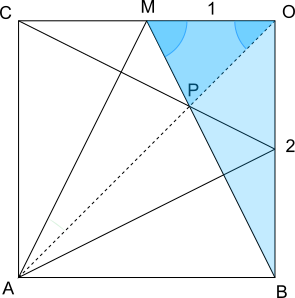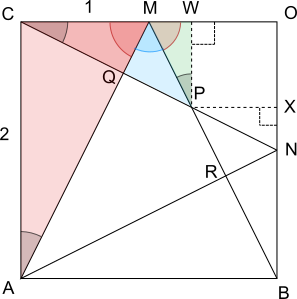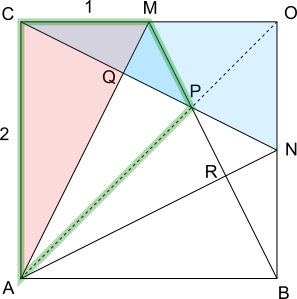#### You may also like### Golden Thoughts

Rectangle PQRS has X and Y on the edges. Triangles PQY, YRX and XSP have equal areas. Prove X and Y divide the sides of PQRS in the golden ratio.### At a Glance

The area of a regular pentagon looks about twice as a big as the pentangle star drawn within it. Is it?### Contact

A circular plate rolls in contact with the sides of a rectangular tray. How much of its circumference comes into contact with the sides of the tray when it rolls around one circuit?

# From All Corners

##### Age 14 to 16Challenge Level
Straight lines are drawn from each corner of a square to the mid points of the sides which are opposite, as shown.An octagon is formed by the eight lines.
Can you work out the area of the octagon as a fraction of the area of the square?

Below are four different methods for finding the area of the octagon as a fraction of the area of the square. Unfortunately, the statements have been muddled up. Can you put them in the correct order?

Using the sine rule and trigonometry for the area of a triangle(A) Apply the sine rule to triangle $OMP$:

(B) Consider just the square in the bottom left-hand quarter of the diagram. Assume that its sides are 2 units long. The diagonal lines coming from the corners will still go through the midpoints of the sides. Line $AO$ has been added, and it is a line of symmetry.

(C) Angle $M\hat{O}P = 45^\circ$, and so angle $O\hat{P}M = 180^\circ-45^\circ-63.43...=71.56...$

(D) Triangle $OBM$ is right-angled with sides of length 1 and 2, and contains angle $O\hat{M}B$, so $O\hat{M}B=\text{tan}^{-1}\left(\frac21\right) = 63.43...^\circ$

(E) The area of triangle $OMP$ can be found using $\frac12ab\sin{C}$:

(F) Since the area of the whole square is $4$, and the area of each half of the part of the octagon is $\frac1 3$, the octagon occupies $\dfrac{\frac23}4 = \dfrac{\frac13}2=\frac16$ of the area of the square.

(J) $\dfrac {MP}{\sin{45}} = \dfrac{1}{\sin{71.56...}} \Rightarrow MP=\dfrac {\sin{45}}{\sin{71.56}} = 0.74...$ or $\frac{\sqrt5}{3}$

(K) $\frac12\times 1 \times \frac {\sqrt 5}{3} \times \sin{63.43...} = \frac 1 3$

Using coordinate geometry (straight line equations)(A) P lies on $L$, so $b=-2a+1$

(B) Line $M$ has gradient $-\frac12$

(C) Put the origin (0,0) at the centre of the diagram, and let the top right-hand corner of the square be at (1,1). Consider only the part of the diagram that lies in the first quadrant.

(D) $\left(\frac13\right)^2 + \frac12\times\left(\frac13\right)\left(\frac12-\frac13\right)\times2$
$=\frac19 + \left(\frac13\right)\left(\frac12-\frac13\right)$
$=\frac19 + \frac13 \times \frac16$
$=\frac19+\frac1{18}$
$=\frac3{18}=\frac16$

(E) Let the lines drawn to the midpoints (0,1) and (1,0) be $L$ and $M$ respectively. $L$ meets the vertex at (1,-1), so it also goes through the point ($\frac12$,0) and similarly $M$ goes through the point (0,$\frac12$). $L$ and $M$ meet at P, with coordinates ($a$, $b$).

(F) Line $L$ has equation $y=-2x+1$

(G) $b = - 2\times\frac13 + 1 =\frac13$

(H) Since the total area of this quarter of the diagram is $1$, the fraction of the area occupied by the octagon is $\frac16$.

(I) Line $L$ has gradient $-2$

(J) $-2a+1=-\frac12a+\frac12$
$\Rightarrow -4a + 2 = -a+1$
$\Rightarrow 1 = 3a$
$\Rightarrow a=\frac13$

(K) Line $M$ has $y$ intercept $\frac12$

(L) P lies on $M$, so $b=-\frac12a+\frac12$

(M) The area inside the octagon is equal to the area of the two small right-angled triangles plus the area of the square. That is equal to:

(N) Line $L$ has $y$ intercept $1$

(O) Line $M$ has equation $y=-\frac12x+\frac12$

Using classical trigonometry only(A) Since the angles in triangle $ACM$ add up to $180^\circ$, angle $A\hat{M}C = 90 - 26.56... = 63.43...^\circ$

(B) $\Rightarrow \frac35\times MP = \frac{1}{\sqrt5}$
$\Rightarrow MP = \frac {\sqrt5}{3}$ or $0.745...$

(C) Angle $M\hat{A}C = \text{tan}^{-1}\left(\frac12\right)=26.56...^\circ$

(D) Consider just the square in the bottom left-hand quarter of the diagram. Assume that its sides are 2 units long. The diagonal lines coming from the corners will still go through the midpoints of the sides. Points $W$ and $X$ have been added where vertical and horizontal lines from $P$ meet the edges of the diagram.

(E) The angles in triangle $CMQ$ are equal to the angles in triangle $ACM$, so triangle $CQM$ is similar to triangle $ACM$, and $AM$ and $CN$ are perpendicular

(F) The area of square $OWPX$ is $\left(\frac23\right)^2 = \frac49$, so the area of the part of the diagram that is in the octagon is $\frac69=\frac23$

(G) Triangle $ACM$ is congruent to triangle $CON$, so angles $N\hat{C}O = Q\hat{C}M$ and $M\hat{A}C$ are equal

(H) From triangle $MPQ$, $\cos{53.13...}=\frac{MQ}{MP}$

(I) So $MW = \frac13$ and $WP = \frac23$

(J) So the hypotenuse of triangle $MPW$ is $\frac{\sqrt 5}{3}$ or $0.745...$

(K) The angles on the line $CO$ at point $M$ add up to $180^\circ$, so angle $A\hat{M}B = 180^\circ - 2\times 63.43...^\circ = 53.13...^\circ$

(L) The total area of this diagram is $2\times2=4$, so the fraction that the octagon occupies is $\dfrac{\frac23}{4} = \dfrac16$

(M) So the scale factor from triangle $ACM$ to triangle $MPW$ is $\frac13$

(N) From triangle $CMQ$, $\sin{26.56...}=\frac{MQ}{1} \Rightarrow MQ = \frac{1}{\sqrt5}$ or $0.447...$

(O) The area of triangle $MPW$ is $\frac12\times\frac13\times\frac23 = \frac19$, so the areas of triangles $MPW$ and $NPX$ add up to $\frac29$

(P) Triangle $MPW$ is similar to triangle $ACM$, and the hypotenuse of triangle $ACM$ can be found using Pythagoras' Theorem : $1^2 + 2^2 = 5$ so $AM=\sqrt5$

(Q) Triangle $MBO$ is congruent to triangle $ACM$, so angle $B\hat{M}O = P\hat{M}W =63.43...^\circ$

Using Pythagoras' Theorem only(A) Consider just the square in the bottom left-hand quarter of the diagram. Assume that its sides are 2 units long. The diagonal lines coming from the corners will still go through the midpoints of the sides. Line $AO$ has been added, and it is a line of symmetry.

(B) Applying Pythagoras' Theorem to triangles $ACM$ and $CON$ gives $1^2+2^2 = 5$, so $AM=CN=\sqrt{5}$

(C) Quadrilateral $ACMP$ has perpendicular diagonals, so its area can be found by multiplying its diagonals and dividing by $2$ (since it contains $4$ right-angled triangles). So the area of quadrilateral $ACMP$ is $AM\times CP\div2$

(D) Triangle $ACM$ is right-angled, and so angles $A\hat{M}C$ and $M\hat{A}C$ add up to $90^\circ$

(E) Triangle $ACM$ is congruent to triangle $CON$, so angles $N\hat{C}O = Q\hat{C}M$ and $M\hat{A}C$ are equal

(F) $PM=PN$ by symmetry

(G) Triangle $CQM$ has hypotenuse $1$, so the scale factor from triangle $CQM$ to triangle $ACM$ is $\sqrt5$. So $CQ = \frac2{\sqrt5}$ and $MQ=\frac1{\sqrt5}$ (or $0.894$ and $0.447$)

(H) So the total area of quadrilateral $ACMP$ and quadrilateral $ABNP$ is $\frac{10}3$, and the total area of the diagram is $2\times2 = 4$. So $\dfrac{\frac{10}3}{4} = \dfrac{\frac{20}{6}}{4}=\dfrac{5}{6}$ of the diagram is outside of the octagon, which means the octagon occupies $\frac16$ of the diagram.

(I) So $AM$ and $CN$ are perpendicular, and triangle $CQM$ is similar to triangle $ACM$

(J) $\Rightarrow \left(\frac1{\sqrt5}\right)^2 + QP^2 = PN^2$
$\Rightarrow \frac15 + QP^2 = \left(\frac 3 {\sqrt5} - QP\right)^2$
$\Rightarrow \frac15 + QP^2= \frac95 - \frac 6 {\sqrt5} QP + QP^2$
$\Rightarrow \frac 15 = \frac 95 - \frac 6{\sqrt5} QP$
$\Rightarrow \frac 6 {\sqrt5} QP = \frac 85$
$\Rightarrow 6QP = \frac 85 \times \sqrt5 =\frac{8\sqrt5}5$
$\Rightarrow QP =\frac{8\sqrt5}{5\times6} = \frac {4\sqrt5}{15}$ or $0.596$

(K) $CN = CQ + QP + PN \Rightarrow \sqrt5= \frac2 {\sqrt5} + QP + PN \Rightarrow QP + PN = \frac 3 {\sqrt5}$ or $1.342$, so $PN = \frac 3 {\sqrt5} - QP$ or $1.342-QP$

(L) $= \sqrt5 \times \left( \frac2{\sqrt5} + \frac {4\sqrt5}{15}\right)\div 2$
$= \left( 2 + \frac {4\times5}{15} \right)\div2$
$= \left( 2 + \frac 4 3\right)\div 2$
$= \frac {10}3\div2$
$= \frac 53$

(M) Therefore angles $Q\hat{C}M$ and $C\hat{M}Q$ add up to $90^\circ$, and so triangle $CQM$ is right-angled

(N)  Applying Pythagoras' Theorem to triangle $MPQ$ gives $MQ^2 + QP^2 = PM^2$